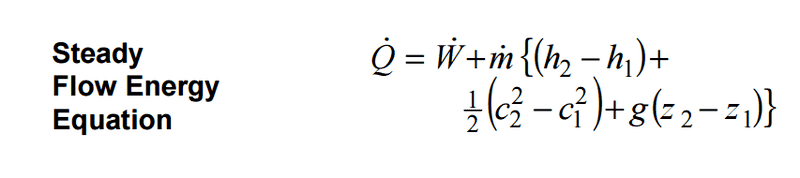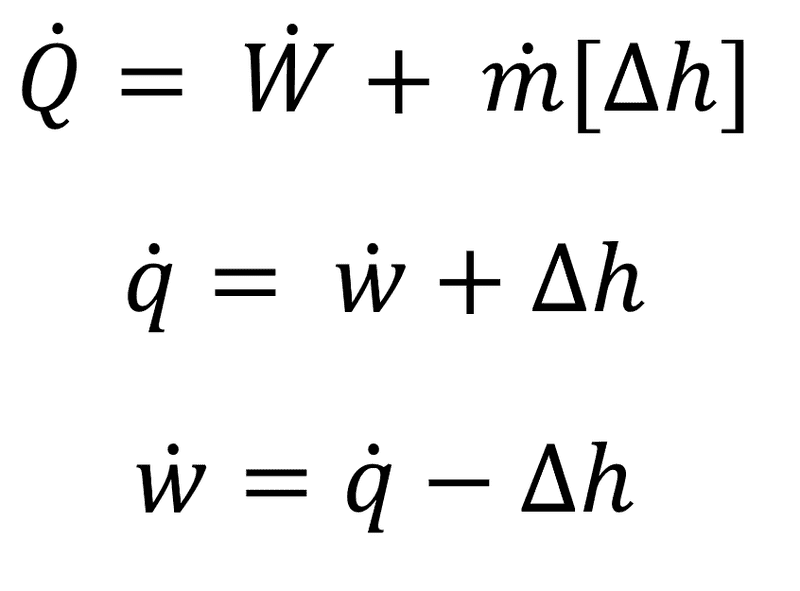# Question about where a thermodynamics formula comes from

• influx
The laws of thermodynamics define how we can relate the desired macroscopic parameters or observables of the system, and in the open system version of the first law, the rate of doing work is only for "shaft work" and not for work involved in pushing mass in and out of the control volume. This explains why the equation includes Δh instead of Δu.

## Homework Statement

How do we obtain:
w = q - Δh

## Homework Equations## The Attempt at a Solution

If you neglect the second half of the SFEE and simplify we get:What is the next step from the last line in the above image to

w = q - Δh

I mean dw/dt = dq/dt - Δh

so if we multiply by dt we get:

dw = dq - Δh(dt)
w = q -
Then I assume this should be integrated? If yes, how? Can't seem to get my head round it.

Also, what is the difference between w = q - Δh and w = q - Δu? I know u is specific internal energy and h is specific enthalpy but the above seems to suggest they're the same?

Last edited:
influx said:
How do we obtain:
w = q - Δh
in thermodynamics, the thermodynamic relation is generally expressed as an infinitesimal change in internal energy in terms of infinitesimal changes in entropy, and volume for a closed system in thermal equilibrium in the following ...
however the laws of thermodynamics predefines the way one can relate the 'desired macroscopic parameters/observables of the system'

In the open system version of the 1st law that you have written (based on a fixed control volume), ##\dot{W}## is not the total rate of doing work, it is only the rate of doing "shaft work." There is also work involved in pushing mass out of the control volume in an output stream, and pushing work into the control volume in an input stream. That explains why there is a ##\Delta h## and not a ##\Delta u##. This should all have been explained in the derivation in your book.

Chet